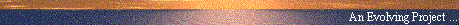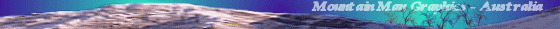# What is the shapeof a falling Raindrop?

#### by A. Resnick (sci.physics)

##### Web Publication by Mountain Man Graphics, Australia# What is the shape of a falling Raindrop?

Date: Tue, 01 Sep 1998 08:54:01 -0500
From: Andy Resnick
Newsgroups: sci.physics
Subject: Re: Raindrop

```

arron wrote:

> What is the shape of a falling Raindrop?

Arron,

The quick answer is 'roughly spherical'.

Here is a long, drawn-out answer.  The static shape of a fluid drop,
jet, bridge, or film is determined by a dimensionless number called the
Bond number.  (Note the contact angle is important, too)  The Bond
number is the ratio of gravitational force to surface tension force.
Thus, a large drop, jet, or bridge will look the same as a small one
provided the Bond numbers for the two are the same.  Two possible static
drop configurations are the 'pendant' and 'sessile' drop shapes
(consider a drop in zero-gravity a limit of a pendant drop as either the
radius of the drop goes to infinity or the radius of the support goes to
zero), and these are easily observed: raindrops on a freshly-waxed car
is a typical sessile drop shape.

Now for dynamics: the basic problem is that the raindrop has a free
surface. That is, the boundary condition for the flowfield within the
drop depends on the drop shape, but the drop shape is not a given.  In
dynamic situations, the important quantity (in addition to the Bond
number) is the relative viscosities of the two fluids.  Usually, it is
assumed that the drop/bridge/jet is axisymmetric, unless there is a good
reason to do otherwise.   The thing the remember is that large drops and
small drops look the same, given identical Bond numbers and relative
viscosities.

It is very difficult to model the process of a drop pinching off from a
jet because the topological qualities of the flow change: we start with
one continuous fluid body, and then undergo a non-reversable
transformation to two disconnected fluid bodies, picking up a
singularity or two along the way.  However, we can do a few things by
considering the drop to have a static shape, and then subject it to a
uniform axially-directed flow. Then we have an iterated process:
calculate the induced surface flow -> calculate the bulk flow ->
calculate the shape change -> calculate the surface flow -> etc...  It's
done by balancing forces, basic Newtonian physics,  but it's rather
tedious. I know of one calculation done for the purposes of modeling
ink-jet printers:

Fromm, J. E. (1984), Numerical calculation of the fluid dynamics of
drop-on demand jets, IBM Journal Research Dev. vol 28, p 322.

And a paper about two drops interacting in a dispersion, either
side-by-side or motion in a row:

Lanbein, D., (1993), Theoretical Aspects of Particle Interactions in
Dispersions, Advances in Colloid and Interface Science, vol 46, p 91.

Like I first said, the drop shape is roughly spherical.  It is not like
most artist renderings of some sort of exaggerated pendant shape.

Andy Resnick

```Usenet Index | Mountain Man Graphics | E-Mail